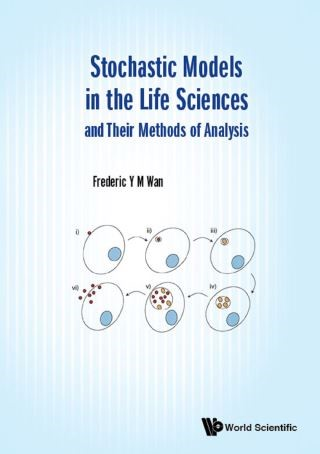## Stochastic Models in the Life Sciences and Their Methods of Analysis• Description
• Author
• Info
• Reviews

### Description

Biological processes are evolutionary in nature and often evolve in a noisy environment or in the presence of uncertainty. Such evolving phenomena are necessarily modeled mathematically by stochastic differential/difference equations (SDE), which have been recognized as essential for a true understanding of many biological phenomena. Yet, there is a dearth of teaching material in this area for interested students and researchers, notwithstanding the addition of some recent texts on stochastic modelling in the life sciences. The reason may well be the demanding mathematical pre-requisites needed to 'solve' SDE.

A principal goal of this volume is to provide a working knowledge of SDE based on the premise that familiarity with the basic elements of a stochastic calculus for random processes is unavoidable. Through some SDE models of familiar biological phenomena, we show how stochastic methods developed for other areas of science and engineering are also useful in the life sciences. In the process, the volume introduces to biologists a collection of analytical and computational methods for research and applications in this emerging area of life science. The additions broaden the available tools for SDE models for biologists that have been limited by and large to stochastic simulations.

Contents:
• Discrete Stage Markov Chains:
• Discrete Sample Space Probability
• Discrete Stage Regular Markov Chains
• Discrete Stage Absorbing Markov Chains
• Discrete Stage Nonlinear Markov Processes
• Continuous Time Markov Chains:
• Continuous Time Birth and Death Type Processes
• Spread of Chlamydia and Stochastic Optimization
• Random Walk, Diffusion and Heat Conduction
• Continuous State Random Variables:
• Continuous Sample Space Probability
• Transformations and Stochastic ODE
• Continuous Stochastic Processes
• Stochastic Ordinary Differential Equations:
• Linear ODE with Random Forcing
• General Nonlinear ODE Systems
• Stochastic Partial Differential Equations:
• Linear PDE with Random Forcing
• Dpp Gradient in the Wing Imaginal Disc of Drosophila
• First Exit Time Statistics:
• First Exit Time
• The Hodgkin–Huxley Model Neuron
• Numerical Simulations

Readership: Undergraduates, graduates, research students, professionals with interests in mathematical biology, stochastic processes and infectious diseases.Mathematical Modeling;Stochastic Models;Stochastic Methods;Mathematical Biology;Life Sciences;Probabilistic Methods;Stochastic Processes;Stochastic Differential Equations;Markov Chains;Markov Processes00